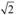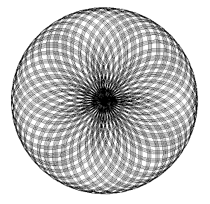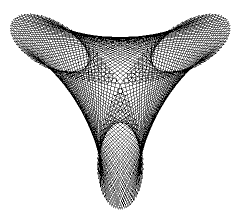deutsch     english    français     Print

## 3.12 PRINTING IMAGES### INTRODUCTION

 You have already learned how to let the turtle draw on a high resolution printer in the chapter Turtle Graphics. You can similarly render an image from GPanel on the printer. You can also use a virtual printer that creates a graphic file in high resolution format (such as Tiff or EPS) [more... For example Image Printer]. To do this, you define a parameterless function with any name such as doIt() that will contain all of the commands necessary to create the image. With a direct call, the image will appear on the screen. To print it, call printerPlot(doIt). You can also specify a scaling factor k, and if you do, call printerPlot(doIt, k) instead. If k < 1 it results in a reduction, and if k >1 it results in an enlargement. PROGRAMMING CONCEPTS: High resolution graphic

### ROSETTES

 The rose-like curves go all the way back to the 18th century to the mathematician Guido Grandi [more... They are also called Rhodonea curves] The generating functions are most easily expressed using polar coordinates (ρ, φ). It has a parameter n:                   ρ = sin(nφ)  The Cartesian coordinates are obtained as usual:                   x =  ρ cos(φ)                   y =  ρ sin(φ) You get a pretty rosette using n =. However, it looks even nicer on a printer than it does on the screen.```from gpanel import *
import math

def rho(phi):
return math.sin(n * phi)

def doIt():
phi = 0
while phi < nbTurns * math.pi:
r = rho(phi)
x = r * math.cos(phi)
y = r * math.sin(phi)
if phi == 0:
move(x, y)
else:
draw(x, y)
phi += dphi

n = math.sqrt(2)
dphi = 0.01
nbTurns = 100
makeGPanel(-1.2, 1.2, -1.2, 1.2)
doIt()
printerPlot(doIt)
```
Highlight program code (Ctrl+C copy, Ctrl+V paste)

### MEMO

 Depending on the choice of the parameter n, you can create different kinds of curves. Try it with natural numbers, rational numbers (fractions), and with irrational numbers (π, e).

### MAURER ROSES

 The mathematician Peter Maurer introduced these curves in 1987 in his article "A Rose is a Rose...". They use rosettes as "guidelines". From this guideline you repeatedly choose points, after a specific rotation angle d, 360 points in total. Afterwards, you connect these points with straight lines. Depending on the choice of n and d, completely different curve shapes can be created. Print them to make them look even nicer (in this example, n = 3 and d = 47 degrees).```from gpanel import *
import math

def sin(x):
return math.sin(math.radians(x))

def cos(x):
return math.cos(math.radians(x))

def cartesian(polar):
return [polar * cos(polar), polar * sin(polar)]

def rho(phi):
return sin(n * phi)

def doIt():
for i in range(361):
k = i * d
pt = [rho(k), k]
corners.append(pt)

move(cartesian(corners))
for pt in corners:
draw(cartesian(pt))

corners = []
n = 3
d = 47
makeGPanel(-1.2, 1.2, -1.2, 1.2)
doIt()
printerPlot(doIt)
```
Highlight program code (Ctrl+C copy, Ctrl+V paste)

### MEMO

In the program you use degrees, not radians. Therefore, it is convenient to define your own functions for sine and cosine that calculate with degrees. This also simplifies the notation, since you will not always need to write math. beforehand.

Likewise, it is convenient to make a conversion from polar to Cartesian coordinates in the function cartesian(), where the coordinate pairs are packaged as a list.

Save the polar coordinates of the 361 points which you select from the guideline in the list corners. In the end, you run through them and draw lines from point to point using draw().
You can draw other known Maurer roses with the following parameters:

 n d 2 39 2 31 6 71

### EXERCISES

 1 Draw 50 concentric circles with the function wave(center, wavelength) with center as the midpoint and wavelength as the radius increment. One could interpret the image as the peaks of a circular wave. Draw the wave with a slightly displaced center and then look at the resulting interference image on a printout. What curve known from geometry can you recognize?